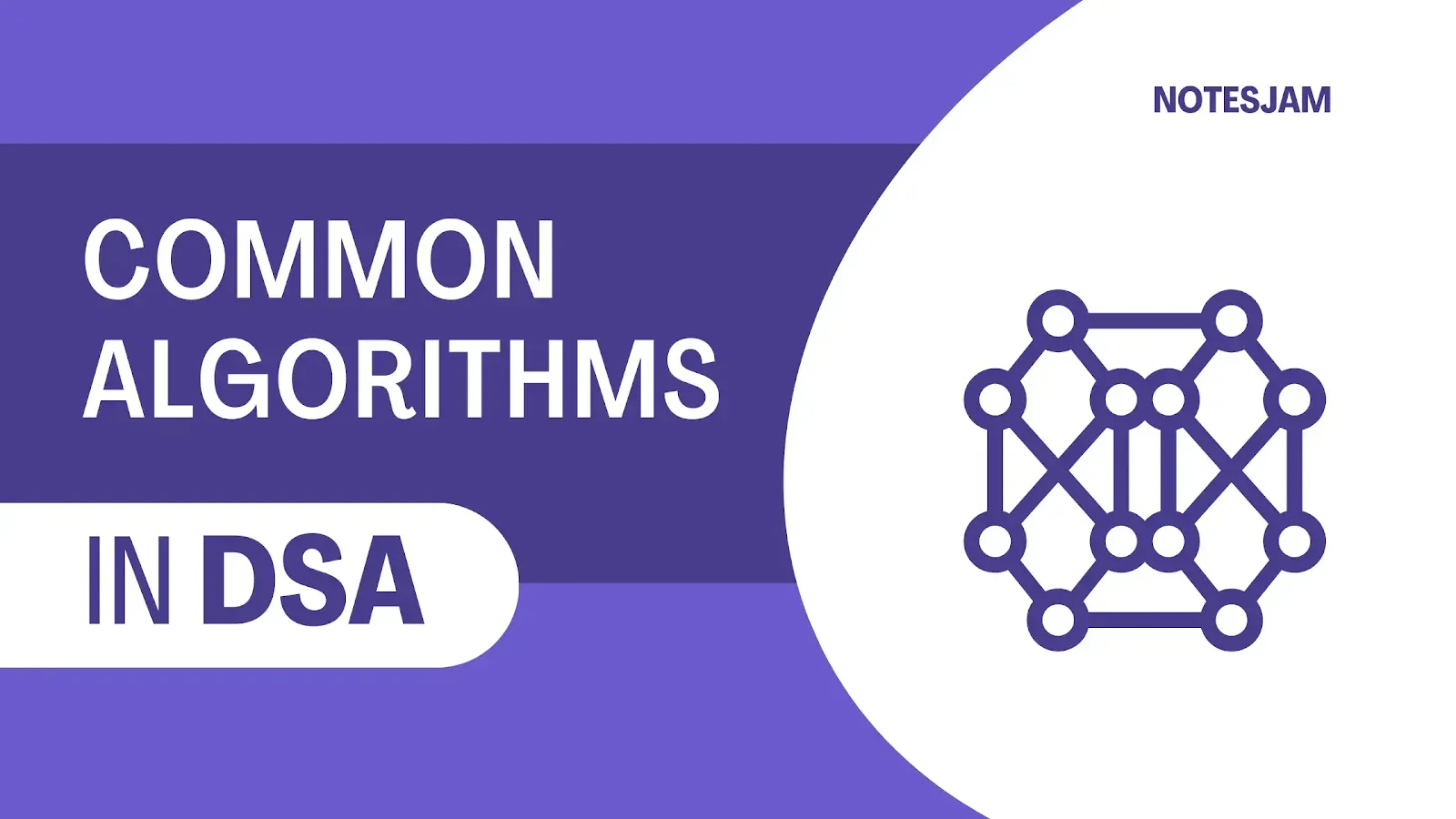# Fundamental Algorithms Every Computer Science Student Should KnowSave important notes and articles from internet using arusaquotes app.

Algorithms are essential tools for solving problems in computer science and related fields. They are the building blocks of software development and play a crucial role in the performance and efficiency of software systems.

In this beginner-friendly tutorial, we'll introduce you to the most common algorithms used in programming, including sorting, searching, graph algorithms, and machine learning. We'll explain each algorithm's purpose and provide examples to help you get started.

By the end of this post, you'll have a solid understanding of these fundamental algorithms and be better equipped to tackle programming challenges.

## Sorting Algorithms

Sorting algorithms are used to arrange data in a particular order. There are many sorting algorithms, but some of the most common are:

Bubble Sort: Bubble sort is a simple sorting algorithm that repeatedly steps through the list, compares adjacent elements and swaps them if they are in the wrong order.

Selection Sort: Selection sort is a simple sorting algorithm that sorts an array by repeatedly finding the minimum element from the unsorted part of the array and putting it at the beginning.

Insertion Sort: Insertion sort is a simple sorting algorithm that builds the final sorted array one item at a time. It is much less efficient on large lists than more advanced algorithms such as quicksort, heapsort, or merge sort.

Quick Sort: Quick sort is a widely used sorting algorithm that uses a divide-and-conquer approach. It picks an element as a pivot and partitions the array around the pivot.

## Searching Algorithms

Searching algorithms are used to find a specific value or element in a collection of data. Some of the most common searching algorithms are:

Linear Search: Linear search is a simple searching algorithm that searches for an element by checking each element in the list in order until it is found.

Binary Search: Binary search is a searching algorithm that is used to search a sorted array by repeatedly dividing the search interval in half.

## Graph Algorithms

Graph algorithms are used to solve problems related to graphs, which are a collection of vertices or nodes and edges that connect them. Some of the most common graph algorithms are:

Breadth-First Search: Breadth-first search is a graph traversal algorithm that starts at the root node and explores all the neighboring nodes at the current depth before moving on to the next level.

Depth-First Search: Depth-first search is a graph traversal algorithm that starts at the root node and explores as far as possible along each branch before backtracking.

Dijkstra's Algorithm: Dijkstra's algorithm is a shortest path algorithm that finds the shortest path between a source node and all other nodes in a graph.

### Dynamic Programming Algorithms

Dynamic programming algorithms are used to solve problems by breaking them down into smaller subproblems and solving them in a recursive manner. Some of the most common dynamic programming algorithms are:

Fibonacci Series: Fibonacci series is a sequence of numbers in which each number is the sum of the two preceding ones. It is a classic example of a dynamic programming problem.

Knapsack Problem: The knapsack problem is a problem in combinatorial optimization that is used to find the maximum value that can be obtained by filling a knapsack with a certain weight limit.

## Machine Learning Algorithms

Machine learning algorithms are used to analyze data and make predictions or decisions based on that data. Some of the most common machine learning algorithms are:

Linear Regression: Linear regression is a statistical method that is used to model the relationship between two variables by fitting a linear equation to the data.

Logistic Regression: Logistic regression is a statistical method that is used to analyze the relationship between a dependent variable and one or more independent variables.

Decision Trees: Decision trees are a type of supervised learning algorithm that is used for classification and regression analysis.

## Conclusion

In conclusion, understanding these common algorithms can help you write efficient and performant code. While this list is not exhaustive, it is a good starting point for software developers looking to improve their algorithmic skills.

Remember that there are always trade-offs when selecting an algorithm for a particular problem, and choosing the right one requires careful analysis of the problem's characteristics and requirements.